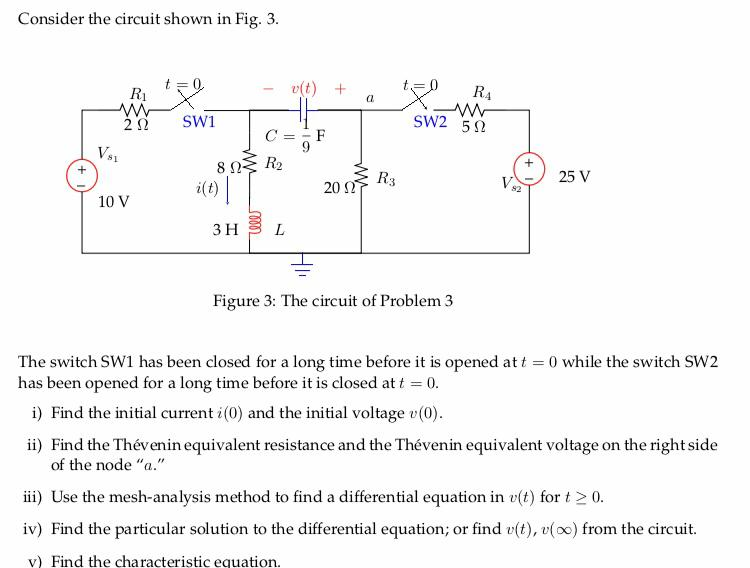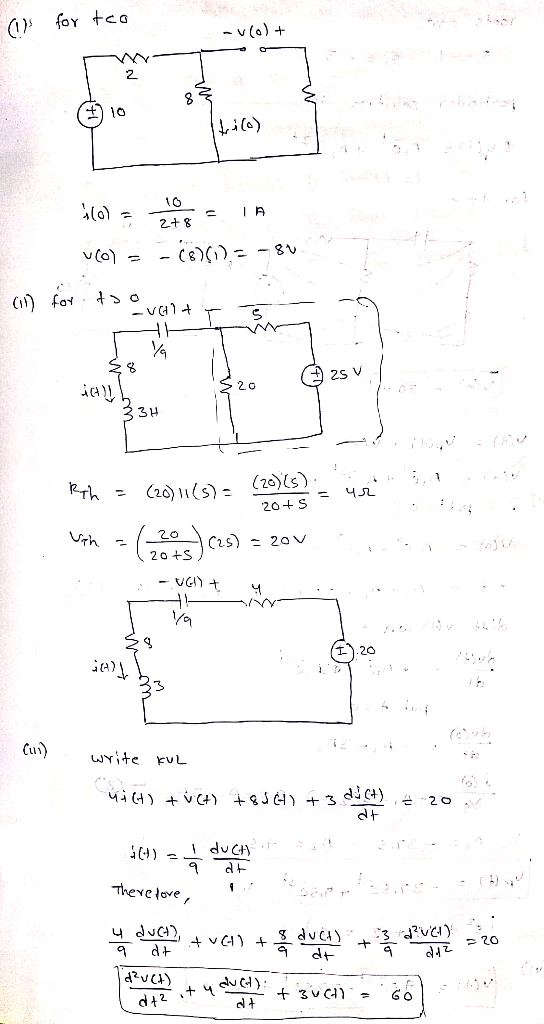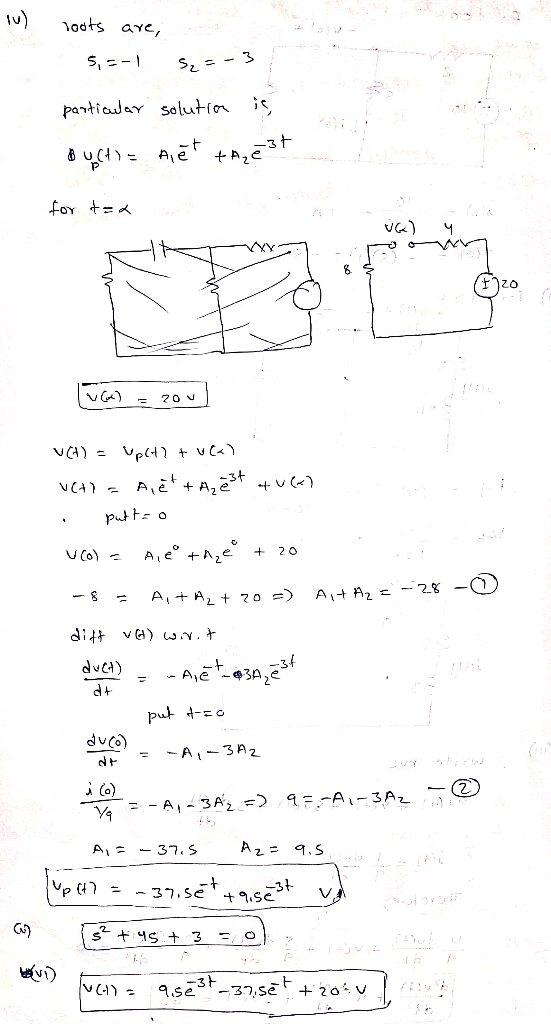# Don't use Laplace method Don't use Laplace method Don't use Laplace method Don't use Laplace meth...Don't use Laplace method

Don't use Laplace method

Don't use Laplace method

Don't use Laplace method

Don't use Laplace method

Consider the circuit shown in Fig. 3 22 SW1 SW2 5Ω CF 25 V 20 S 10 V 3 H BL Figure 3: The circuit of Problem3 The switch SW1 has been closed for a long time before it is opened att has been opened for a long time before it is closed att 0 0 while the switch SW2 i) Find the initial current i(0) and the initial voltage (0) ii) Find the Thévenin equivalent resistance and the Thévenin equivalent voltage on the rightside of the node"a" ii) Use the mesh-analysis method to find a differential equation in v(t) fort 2 0 iv) Find the particular solution to the differential equation; or find (t), voo) from the circuit. v Find the characteristic equation.
vi) Find the solution of the differential equation, t), fort20.##### Add Answer of: Don't use Laplace method Don't use Laplace method Don't use Laplace method Don't use Laplace meth...
More Homework Help Questions Additional questions in this topic.

• #### For each of the following foreign bodies identify the type of cell that ingests them and the meth...

Need Online Homework Help?